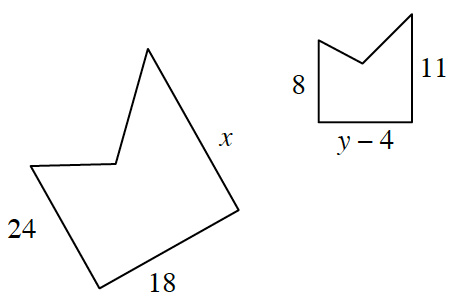### Home > INT2 > Chapter 3 > Lesson 3.1.5 > Problem3-65

3-65.

Use what you know about a scale factor between similar figures to answer the following questions.

1. Assume a triangle has side lengths $6, 7,$ and $10$ units while another triangle has side lengths $3, 4,$ and $5$ units. Are these triangles similar? How do you know?

Does each side of the first triangle have the same scale factor to the
corresponding sides of second triangle?

No, because $3(2) = 6$ and $5(2) = 10$, but $4\left(2\right)$ does not equal $7$. The corresponding
side lengths are not all multiplied by the same scale factor.

2. The pentagons at right are similar. Use what you have learned about similarity to write equations and solve for the values of $x$ and $y$.

Use these proportions to solve for the missing variables.

$\frac{24}{8} \ = \ \frac{x}{11}$

$\frac{18}{y - 4} \ = \ \frac{24}{8}$

$x = 33$ and $y = 10$

3. Give a possible sequence of transformations that would map the pentagon on the left onto the pentagon on the right.

Think about ways to rotate the shape and change it to match the other shape.

Translate any vertex to its corresponding vertex, rotate so that the angle
sides of that vertex coincide, then dilate the image of the pentagon through the
vertex with a scale factor of one-third to reduce it.